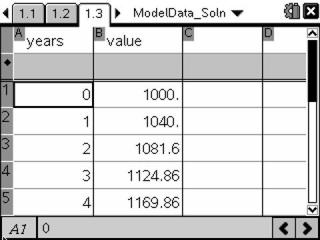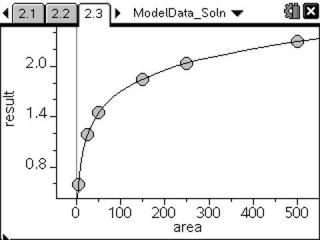# Activities

••• ##### Subject Area

• Math: Algebra II: Logarithms and Exponentials
• Math: Algebra II: Logarithmic Functions
• Math: College Algebra: Exponential and Logarithmic Functions

• ##### Author9-12

20 Minutes

• ##### Device
• TI-Nspire™ Navigator™
• TI-Nspire™
• TI-Nspire™ CAS
• ##### Software

TI-Nspire™
TI-Nspire™ CAS

2.0

## Modeling Data#### Activity Overview

In this activity, students will graph data modeling exponential and logarithmic growth and find equations representing the data.

#### Key Steps

•In Problem 1 of this activity, students will find the interest rate for given data to determine an interest rate equation.

•In Problem 2 of this activity, students will determine the base for a logarithmic function that models the given data.